Published: 08 December 2016

# An approach to fault diagnosis for gearbox based on order tracking and extreme learning machine

Hua Su1
Chen Lu2
Jian Ma3
1, 2, 3School of Reliability and Systems Engineering, Beihang University, Beijing, China
1, 2, 3Science and Technology on Reliability and Environmental Engineering Laboratory, Beijing, China
Corresponding Author:
Jian Ma
Views 126

#### Abstract

Varying speed machinery condition detection and fault diagnosis are more difficult due to non-stationary machine dynamics and vibration. In this paper, an intelligent fault diagnosis method based on order analysis and extreme learning machine (ELM) is proposed. Order tracking, easily identifying speed-related vibrations, is useful for machine condition monitoring, which could obtain the resampling signal of constant increment angle. Then, the power spectrum (PS) of characteristic orders, as the fault feature vectors, is extracted and normalized from the de-noising signal. Last, in order to diagnose the faults of the gearbox automatically, ELM, provided better generalization performance at a much faster learning speed and with least human intervene, is applied to identify and classify the faults. From the result of experiment, the approach of this paper is effective to judge the fault type under variable speed conditions.

## 1. Introduction

Gearboxes, widely used in machinery equipment, plays a significant role in industrial applications, which are mainly reflected on the large-scale machinery, such as cars , aircraft , wind power generation system . Thus, the gearbox damaged, will result in loss of production and raise the cost of machines’ maintenance . So, it is very important to monitor the condition of the gearboxes to avoid run time breakdown of the machine. However, gearbox is a very complex mechanical system that can generate vibrations from its various elements such as gears, shafts, and bearings . Even though several techniques have been proposed in the literature for feature extraction, such as, time analysis, frequency analysis, wavelet transform (WT), empirical mode decomposition (EMD) and Hilbert transformation method [5-8], it still remains a challenge in implementing a diagnostic tool for real-world monitoring applications because of non-stationary signals in the time domain under the complexity of machinery structures and operating conditions.

The order analysis, a method which can transform non-stationary signals in the time domain into the stationary signal in the angular domain, easily identify speed-related vibrations. K. R. Fyfe et al. introduced two approaches to obtain signals in the angular domain, namely the hard order tracking and computed order tracking (COT), and the comparison of two methods in details is in the Ref. . After that, Vold and Leuridan put forward an order tracking algorithm based on Kalman filtering, which solves interference during orders . And the instantaneous frequency (IF) of a signal is also applied order analysis that it could achieve order analysis without tachometer . In this study, COT is selected as the method of processing signal because of low computational cost and high precision of angular signals.

ELM, originally developed for the SLFNs, provides better generalization performance at a much faster learning speed and with least human intervene . Compared with those traditional computational intelligence techniques, ELM shown its good performance in regression applications as well as in large dataset and multi-label classification applications.

This paper is organized as follows: Section 2 introduces COT and ELM, Section 3 describes the results performed to validate the method and Section 4 presents the conclusions and related future works.

## 2. Methodology

In the paper, a novel approach of the fault pattern recognition is presented, which contains the following steps: (1) Angular transformation of the vibration signal based on computing order tracking; (2) Feature extraction of the angular signals; and (3) Fault diagnosis based on ELM as illustrated in Fig. 1.

### 2.1. Computed order tracking

A method, obtaining the signal in the angular domain based on COT, depends on the rotational speed pulse (see Fig. 2). Compared with the hard order tracking, installing complex devices, COT can obtained rotational speed to install a tachometer to obtain resample signals. After resampling, the signal in angular domain, carried on the Fourier transform, then obtains the order domain. Sampling rate ${O}_{S}$ should meet the conditions of Eq. (1) because of the Nyquist sampling theorem:

1
${O}_{max}\le {O}_{nyquist}=\frac{{O}_{S}}{2},$

where ${O}_{max}$, the maximum analysis order. And in order to ensure a full period sampling, the sampling number of each cycle should be ${2}^{n}$, $n$ equals 1, 2, 3….

Fig. 1The flow chart of the article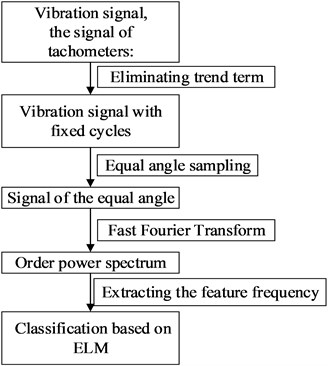Fig. 2Rotational speed pulse signal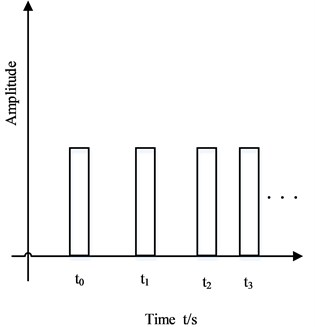Angular resampling is the most important step in the realization of order tracking.

The steps of order tracking methods analysis :

1) Function $n\left(t\right)$ fitted by keyphasor signal.

2) Calculating the angle function $\theta \left(t\right)=\frac{\pi }{30}{\int }_{0}^{t}n\left(t\right)$ of the reference axis.

3) Calculating the resampling time ${t}_{n}$, through the quadratic curve fitting. It is assumed that the shaft is undergoing constant angular acceleration. With this basis, the shaft angle, $\theta$, can be described by a quadratic equation of the following form:

2
$\theta \left(t\right)={b}_{0}+{b}_{1}t+{b}_{2}{t}^{2}.$

The unknown coefficients ${b}_{0}$, ${b}_{1}$, ${b}_{2}$ are found by fitting three successive keyphasor arrival times $\left({t}_{1},{t}_{2},{t}_{3}$), which occur at known shaft angle increments $\mathrm{\Delta }\varphi =2\pi$

Then, this yields the three following conditions, $\theta \left({t}_{1}\right)=0$; $\theta \left({t}_{2}\right)=\mathrm{\Delta }\varphi$; $\theta \left({t}_{3}\right)=2\mathrm{\Delta }\varphi$:

3
$\left(\begin{array}{c}0\\ \mathrm{\Delta }\varphi \\ 2\mathrm{\Delta }\varphi \end{array}\right)=\left[\begin{array}{ccc}1& {t}_{1}& {t}_{1}^{2}\\ 1& {t}_{2}& {t}_{2}^{2}\\ 1& {t}_{3}& {t}_{3}^{2}\end{array}\right]\left\{\begin{array}{c}{b}_{0}\\ {b}_{1}\\ {b}_{2}\end{array}\right\},$
4
${t}_{n}=\frac{1}{{2b}_{2}}\left[\sqrt{4{b}_{2}\left({\theta }_{k}-{b}_{0}\right)+{b}_{1}^{2}}-{b}_{1}\right].$

In the formula, ${t}_{n}$ is the time when the angle of ${\theta }_{k}$ is turned around.

4) Calculating the vibration data ${x}^{\mathrm{\text{'}}}\left(\mathrm{}{t}_{n}\right)$ of the equivalent angle by interpolation of the original vibration signal $x\left(\mathrm{}{t}_{n}\right)$, and interpolating functions are as follows:

5
$x\left(\mathrm{}{t}_{n}\right)=\sum _{k}x\left(k{t}_{s}\right){h}_{s}\left(k{t}_{s}-{t}_{n}\right),$
6
${h}_{s}\left(t\right)=\mathrm{s}\mathrm{i}\mathrm{n}c\left(\pi {f}_{s}t\right)=\frac{\mathrm{s}\mathrm{i}\mathrm{n}\left(\pi {f}_{s}t\right)}{\pi {f}_{s}t}.$

In the formulas, ${t}_{s}$ denotes the interval of sampling in the time domain, the value of $k$ makes that $k{t}_{s}$ and ${t}_{n}$ are the closest, ${h}_{s}$ is the interpolation filter, ${h}_{s}\left(t\right)$ is the interpolation function.

### 2.2. Extreme learning machine

Single-hidden layer feedforward neural networks (SLFNs) algorithm, the structure shown in Fig. 3, is the basis of ELM. Compared with the traditional SLFNs algorithm, ELM is a novel algorithm of SLFN, which has lots of advantages. Obviously, ELM could randomly produce weight between hidden layer and input layer and the thresholds of hidden layer nodes are also stochastically. Furthermore, while training the network, ELM can avoid to adjust weights or thresholds. Simply speaking, the algorithm only needs to set the number of hidden layer neurons to get the unique optimal solution. And learning pace of ELM is very quick and it shows good generalization performance. As follow, the Eq. (7) is the mathematical model of ELM [12, 13]:

7
${f}_{L}\left(x\right)=\sum _{i=1}^{L}{\beta }_{i}{g}_{i}\left(x\right)=\sum _{i=1}^{L}{\beta }_{i}{G}_{i}\left({a}_{i},\mathrm{}{b}_{i},\mathrm{}x\right),x\in {R}^{d},\mathrm{}\mathrm{}\mathrm{}\mathrm{}\mathrm{}{\beta }_{i}\in {R}^{m}.$

In the Eq. (7), ${g}_{i}$ expresses the output function ${G}_{i}\left({a}_{i},\mathrm{}{b}_{i},\mathrm{}x\right)$ of the $i$th node which belongs to the hidden layer.

Fig. 3Single-hidden layer feedforward network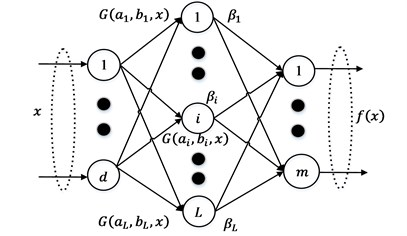For a neural network, the activation function is very important. For ELM, the activation functions of the hidden layer usually have three types:

8
$g\left(x\right)=\frac{1}{\left(1+\mathrm{e}\mathrm{x}\mathrm{p}\left(-a\bullet x+b\right)\right)},$
9
$g\left(x\right)=\left\{\begin{array}{cc}1,& a\bullet x-b\ge 0,\\ 0,& \text{otherwise},\end{array}\right\$
10
$g\left(x\right)={\left({‖x-a‖}^{2}+{b}^{2}\right)}^{1}{2}}.$

In [12, 13], the authors made a statement of advantages and explain that three types of activation functions show good performance. And in this paper, Eq. (8) is selected as the activation function. The steps of ELM algorithm as follows:

1) Determine the number $L$ of neurons in the hidden layer and activation function $g\left(x\right)$, and randomly assign ${a}_{i}$, ${b}_{i}$, ${\beta }_{i}$.

2) Calculate the output vector of $i$th.

3) Calculate the output weight $\stackrel{^}{\beta }$.

## 3. Results

### 3.1. Experimental facilities

The source of the data in this paper comes from the 2009 PHM Conference Data Analysis Competition. The data is about gearbox made of 560 samples which are constituted two types of gearboxes and fourteen kinds of fault modes. And each of the working conditions has different shaft speeds 30, 35, 40, 45, and 50 Hz and four kinds of loads. And the author chooses four typical fault modes (see Table 1) to verify the feasibility of the new algorithm proposed .

Table 1Fault modes chosen of the samples

 Case Normal Fault 1 Fault 2 Fault 3 Gear 32T Good Chipped Good Good 96T Good Good Good Good 48T Good Eccentric Eccentric Eccentric 80T Good Good Good Broken Bearing IS:IS Good Good Good Ball ID:IS Good Good Good Good OS:IS Good Good Good Good IS:OS Good Good Good Good ID:OS Good Good Good Good OS:OS Good Good Good Good Shaft Input Good Good Good Good Output Good Good Good Good

### 3.2. Order tracking and feature extraction

1) Data resampling. In order to reduce system error of the signal, which could be caused by the sensor, signal preprocessing of eliminating trend was implemented. Then COT was used to transform the non-stationary vibration signal of time domain into the stationary signal of angular domain, this paper uses a method named computed order tracking.

Autocorrelation Coefficient (ACF)  is calculated to compare the stability of signals both time domain and angular domain. Autocorrelation Function, effective method to inspect the stability of time series while vibration signal is a kind of time series.

From the Fig. 4, the details of ACFs presented, we can see that the convergence rate of the ACF of signal in the angular domain are faster than the other, which verify the angular signal is more stable than the time signal.

2) Characteristic Parameters Extraction. In this section, characteristic vector was extracted. First, Fast Fourier transform is applied to the angular resampling signal, thus the order power spectrum can be obtained (see Fig. 5). There was six feature order selected as follows: ${W}_{1}$ represents the order power spectrum of order 32; ${W}_{2}$ represents the order power spectrum of order 64; ${W}_{3}$ represents the order power spectrum of order 96; ${W}_{4}$ represents the order power spectrum (PS) of order 128; ${W}_{5}$ represents the order power spectrum of order 48; ${W}_{6}$ represents the order power spectrum of order 80. Then the characteristic parameters of each characteristic order are ${W}_{j}$, $j$ equals 1, 2, 3, 4, 5, 6.

Because of different energy distributions under different operating conditions, it is feasible to use the energy percentage of feature orders as the feature vector. Then the feature parameters are normalized:

11
${\overline{W}}_{j}=\frac{{W}_{j}}{W},T=\left[\begin{array}{cc}\begin{array}{cc}\begin{array}{cc}{\overline{W}}_{1}& {\overline{W}}_{2}\end{array}& \begin{array}{cc}{\overline{W}}_{3}& {\overline{W}}_{4}\end{array}\end{array}& \begin{array}{cc}{\overline{W}}_{5}& {\overline{W}}_{6}\end{array}\end{array}\right],$

$w$, sum of the characteristic parameters ${W}_{j}$, that means $W=\sum _{1}^{6}{W}_{j}$, $j$ equals 1, 2, 3, 4, 5, 6. ${\overline{W}}_{j}$, the normalized parameters of ${W}_{j}$. $T$, the feature vector after normalization processing.

Fig. 4The ACFs of different fault modes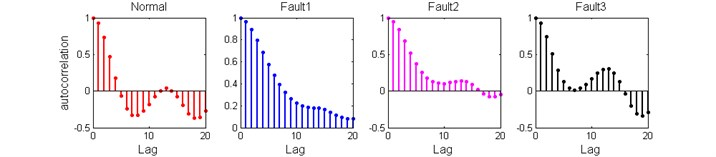a) ACFs in the time domain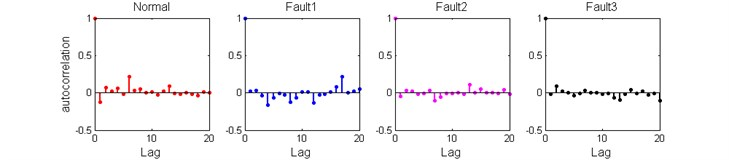b) ACFs in the angular domain

Fig. 5Order power spectrum under different conditions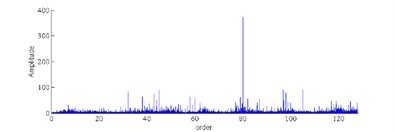a) Normal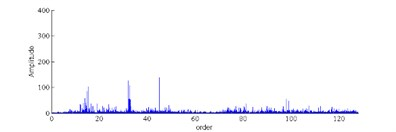b) Fault 1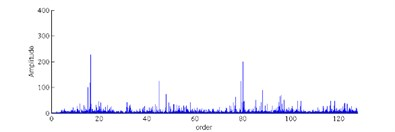c) Fault 2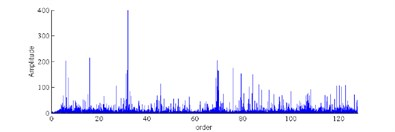d) Fault 3

3) Fault diagnosis based on ELM.

There are 60 samples in each case. 50 samples are used as training samples for the ELM and the rest samples are used as testing samples for the ELM. By the way of cross validation, the classification performance is showed. The final classification accuracy is the average of the results of the 300 ELM’s classification (see Table 2).

Based on the COT-PS-ELM approach, the fault detection results under different loads are studied. The result of fault diagnosis shows on Table 2. From the Table 2, the COT-PS-ELM method shows the accuracies of total are 0.9908, 0.9803, 0.9147, 0.9420 and all of them are greater than 90 %. It is also found that the accuracies of low loads are higher than high loads. Thus, it can be seen COT-PS-ELM is more suitable for low load conditions.

Table 2Accuracy of classification based on COT-PS-ELM

 Load Total Normal Fault 1 Fault 2 Fault 3 Low 1 0.9908 0.9880 0.9850 0.9967 0.9933 Low 2 0.9803 0.9927 0.9777 0.9640 0.9870 High 1 0.9147 0.9430 0.9290 0.8643 0.9227 High 2 0.9420 0.9560 0.9243 0.9417 0.9460

## 4. Conclusions

In this paper, it has been described a new method, COT-PS-ELM, which could be effectiveness to faults diagnosis of gearbox. The COT-PS-ELM scheme is shown to be not sensitive to a particular speed; that is, fault detected with COT-PS-ELM could reduce the effect of different speed operations. Our subsequent work focuses on improving the accuracy of faults diagnosis under the high load conditions.

07 November 2016
Accepted
08 November 2016
Published
08 December 2016
SUBJECTS
Fault diagnosis based on vibration signal analysis
Keywords
order tracking
extreme learning machine
fault diagnosis
Acknowledgements

The authors declare that there is no any potential conflict of interests in the research. This study was supported by the Fundamental Research Funds for the Central Universities (Grant No. YWF-16-BJ-J-18) and the National Natural Science Foundation of China (Grant Nos. 51575021 and 51605014), as well as the Technology Foundation Program of National Defense (Grant No. Z132013B002).

Conflict of interest

The authors declare that there is no potential conflict of interest in this research.